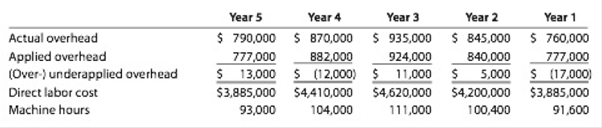Chapter 19, Problem 19.2CP

Chapter
Section
Textbook Problem

Predetermined overhead ratesAs an assistant cost accountant for Mississippi Industries, you have been assigned to review the activity base for the predetermined factory overhead rate. The president, Tony Favre, has expressed concern that the over- or underapplied overhead has fluctuated excessively over the years.An analysis of the company’s operations and use of the current overhead rate (direct labor cost) has narrowed the possible alternative overhead bases to direct labor cost and machine hours. For the past five years, the following data have been gathered:In teams:1. Calculate a predetermined factory overhead rate for each alternative base, assuming that rates would have been determined by relating the total amount of factory overhead for the past five years to the base.2. For each of the past five years, determine the over- or underapplied overhead based on the two predetermined overhead rates developed in part (1).3. Which predetermined overhead rate would you recommend? Discuss the basis for your recommendation.

(1)

To determine

Predetermined factory overhead rate

Normally, factory overhead costs are applied or allocated to cost of job based on predetermined factory overhead rate. The formula to calculate the predetermined factory overhead rate is as follows:

Predetermined factory overhead rate = Estimated total factory overhead costsEstimated activity rate

To calculate: the predetermined factory overhead rate for each alternative base for M Industries.

Explanation

The predetermined overhead rate for alternative activity base is prepared as follows:

Direct labor cost:

Predetermined overhead rate = Total overhead cost Table (1)Total direct labor cost Table (2)=$4,200,000$21,000,000=20% of direct labor cost

Machine hours:

Predetermined overhead rate = Total overhead cost Table (1)Total machine hours Table (3)=$4,200,000500,000=$8

(2)

To determine
the over- or under applied overhead based on two predetermined overhead rates.

(3)

To determine

To discuss: in applying the best predetermined overhead rate as computed.

Still sussing out bartleby?

Check out a sample textbook solution.

See a sample solution

The Solution to Your Study Problems

Bartleby provides explanations to thousands of textbook problems written by our experts, many with advanced degrees!

Get Started

It is common for long-term asset accounts to show a decrease in the net balance from year to year.

College Accounting, Chapters 1-27 (New in Accounting from Heintz and Parry)

The present value of a perpetuity is equal to the payment on the annuity, PMT, divided by the interest rate, I:...

Fundamentals of Financial Management, Concise Edition (with Thomson ONE - Business School Edition, 1 term (6 months) Printed Access Card) (MindTap Course List)

Why should policymakers care about GDP?

Principles of Macroeconomics (MindTap Course List)